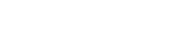# TYPICAL CASES OF AXIALLY LOADED STRUCTURAL MEMBERSReading time: 1 minute

The wire in figure 1 is pulled by the action of a mass attached to its lower end. In this condition the wire is in tension. Suppose the total load on the cross-section of the wire is P and the cross-sectional area of the wire is A, then the uniform tensile stress (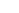) in the wire is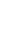. Thisis called the intensity of force P over the cross-sectional areas A.Figure 1

The stress is expressed in terms of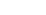or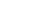or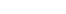etc.

When force P act at each end of a bar towards each other and if they tend to compress the bar, then the forces give rise to compressive stresses.

Tensile and compressive stresses are together referred as direct stresses.

Practical situations of tensile force:

a) Bolt when tightened, the bolt will be subjected to tension.

b) Cable when it sags under its self weight.

Practical situations of compressive stresses:

A column carrying a central axial compressive force.

#### (a) Circular tapering bar subjected to an axial pull (figure 2)Figure 2

Bar of circular cross-section with diameter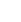at one end and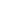at the other end of length L, is subjected to tensile force P.

Elongation over a small length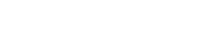Where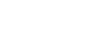Total elongation over the length AB =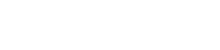#### (b) Square tapering bar subjected to an axial pull (figure 3)

If the bar is of length L and has a square cross-section measuring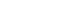at one end and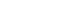at the other end, the total elongation is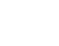#### (c) Bars of varying cross section subjected to an axial pullFigure 3

Axial pull = P

Material of the three parts is not uniform.Total elongation =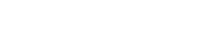In general, total elongation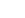is given by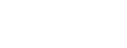#### (d) Bar of uniform strength (figure 4)

Relationship in the variation of cross-section required to ensure that the strength is uniform throughout.Figure 4

Bar is subjected to a tensile load in addition to its own weight

Ifis the stress in bar at any cross-section, then from consideration of an elementary strip dx,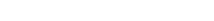Where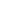is the specific weight of the bar.

In the limit,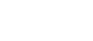Integrating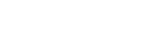or,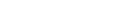when x = L,#### (e) Extension of a bar under its own weight (figure 5)Figure 5

Cross-section (uniform) = A

Consider a small element of length dx.

Extension of the strip =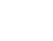Whereis the specific weight of the bar

Total extension of the bar =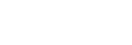If W is the total weight of the bar, then total elongation =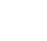It may be observed that the total extension produced by self weight of the bar is equal to that produced by a load of half its weight applied at the lower end.

#### (f) Bar of varying cross-section subjected to a pull due to its self-weight (figure 6)Figure 6

Bar is hung from the top as shown in figure. If A is the variable cross-section, then weight of the bar upto the height x is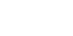Stresses in the strip =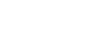Elongation in the strip =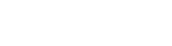Total elongation =